Quantitative MCQ - 15

# Quantitative MCQ - 15

Test Description

## 30 Questions MCQ Test Quantitative Aptitude for Competitive Examinations | Quantitative MCQ - 15

Quantitative MCQ - 15 for Quant 2022 is part of Quantitative Aptitude for Competitive Examinations preparation. The Quantitative MCQ - 15 questions and answers have been prepared according to the Quant exam syllabus.The Quantitative MCQ - 15 MCQs are made for Quant 2022 Exam. Find important definitions, questions, notes, meanings, examples, exercises, MCQs and online tests for Quantitative MCQ - 15 below.
Solutions of Quantitative MCQ - 15 questions in English are available as part of our Quantitative Aptitude for Competitive Examinations for Quant & Quantitative MCQ - 15 solutions in Hindi for Quantitative Aptitude for Competitive Examinations course. Download more important topics, notes, lectures and mock test series for Quant Exam by signing up for free. Attempt Quantitative MCQ - 15 | 30 questions in 30 minutes | Mock test for Quant preparation | Free important questions MCQ to study Quantitative Aptitude for Competitive Examinations for Quant Exam | Download free PDF with solutions
 1 Crore+ students have signed up on EduRev. Have you?
Quantitative MCQ - 15 - Question 1

### Directions (1-5): What should come in place of the question mark (?) in the following questions?  7960+2956-8050+4028= ?

Detailed Solution for Quantitative MCQ - 15 - Question 1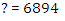Quantitative MCQ - 15 - Question 2

### Directions (1-5): What should come in place of the question mark (?) in the following questions?  25×3.25+50.4÷24= ?

Detailed Solution for Quantitative MCQ - 15 - Question 2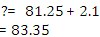Quantitative MCQ - 15 - Question 3

### Directions (1-5): What should come in place of the question mark (?) in the following questions?  350% of ? ÷50+248=591

Detailed Solution for Quantitative MCQ - 15 - Question 3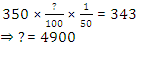Quantitative MCQ - 15 - Question 4

Directions (1-5): What should come in place of the question mark (?) in the following questions?

1/2  of 3842+15% of ?=2449

Detailed Solution for Quantitative MCQ - 15 - Question 4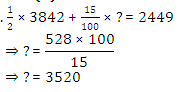Quantitative MCQ - 15 - Question 5

Directions (1-5): What should come in place of the question mark (?) in the following questions?

(833.25-384.45)÷24= ?

Detailed Solution for Quantitative MCQ - 15 - Question 5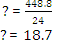Quantitative MCQ - 15 - Question 6

Divide Rs. 1301 between A and B, so that the amount of A after 7 years is equal to the amount of B after 9 years, the interest being compounded at 4% per annum.

Detailed Solution for Quantitative MCQ - 15 - Question 6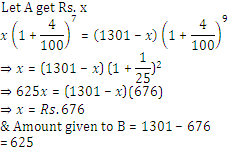Quantitative MCQ - 15 - Question 7

Amit borrowed Rs. 800 at 10% rate of interest. He repaid Rs. 400 at the end of first year. What is the amount he is required to pay at the end of second year to discharge his loan which was calculated at compound interest?

Detailed Solution for Quantitative MCQ - 15 - Question 7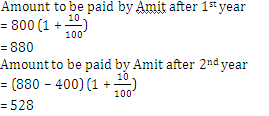Quantitative MCQ - 15 - Question 8

A man borrows Rs. 4000 from a bank at 15/2% compound interest. At the end of every year, he pays Rs. 1500 as part repayment of loan and interest. How much does he still owe to the bank after three such instalments?

Detailed Solution for Quantitative MCQ - 15 - Question 8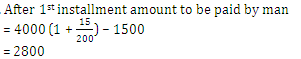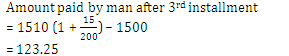Quantitative MCQ - 15 - Question 9

The rates of simple interest in two banks A and B are in the ratio 5 : 4. A person wants to deposit his total savings in two banks in such a way that he receives equal half yearly interest from both. He should deposit the savings in banks A and B in the ratio:

Detailed Solution for Quantitative MCQ - 15 - Question 9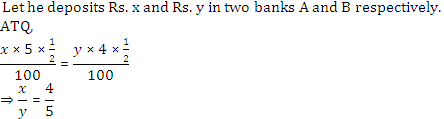Quantitative MCQ - 15 - Question 10

An article costing Rs. 9,000 is sold at a discount which is equal to the simple interest on Rs. 3,000 for N months. Find N if the rate of discount is same as the rate of interest.

Detailed Solution for Quantitative MCQ - 15 - Question 10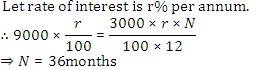Quantitative MCQ - 15 - Question 11

There is a sequence of 11 consecutive odd numbers. If the average of first 7 numbers is X, then find the average of all the 11 integers.

Detailed Solution for Quantitative MCQ - 15 - Question 11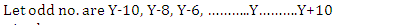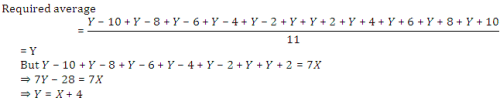Quantitative MCQ - 15 - Question 12

A milkman buys milk contained in 10 vessels of equal size. If he sells his milk at Rs. 5 a litre, he loses Rs. 200; if he sells it at Rs. 6 a litre, he would gain Rs. 150 on the whole. Find the number of litres contained in each vessel:

Detailed Solution for Quantitative MCQ - 15 - Question 12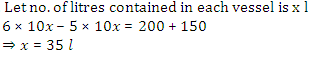Quantitative MCQ - 15 - Question 13

The mean of 25 observations was found to be 78.4. But later on it was found that 96 was misread as 69. The correct mean is:

Detailed Solution for Quantitative MCQ - 15 - Question 13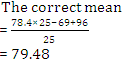Quantitative MCQ - 15 - Question 14

A trader has 50 kg of rice, a part of which he sells at 10% profit and the rest at 5% loss. He gains 7% on the whole. How much was sold at 10% gain and how much was sold at 5% loss?

Detailed Solution for Quantitative MCQ - 15 - Question 14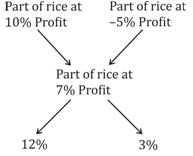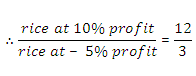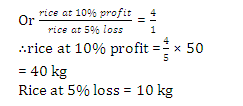Quantitative MCQ - 15 - Question 15

By selling 12 marbles for a rupee, a shopkeeper loses 20% In order to gain 20% in the transaction, he should sell the marbles at the rate of how many marbles for a rupee?

Detailed Solution for Quantitative MCQ - 15 - Question 15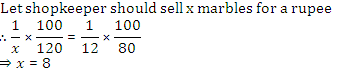Quantitative MCQ - 15 - Question 16

Directions (Q.16-20): What approximate value will come in place of question mark (?) in the following questions? (You are not expected to calculate the exact value.)

(7171+3854+1195)÷(892+214+543)=?

Detailed Solution for Quantitative MCQ - 15 - Question 16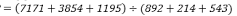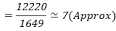Quantitative MCQ - 15 - Question 17

Directions (Q.16-20): What approximate value will come in place of question mark (?) in the following questions? (You are not expected to calculate the exact value.)

69.008% of 699.998+32.99% of 399.999= ?

Detailed Solution for Quantitative MCQ - 15 - Question 17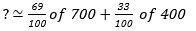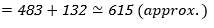Quantitative MCQ - 15 - Question 18

Directions (Q.16-20): What approximate value will come in place of question mark (?) in the following questions? (You are not expected to calculate the exact value.)

( 24.99 )2 + ( 31.05 )2 = (?)2

Detailed Solution for Quantitative MCQ - 15 - Question 18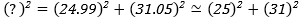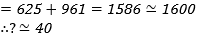Quantitative MCQ - 15 - Question 19

Directions (Q.16-20): What approximate value will come in place of question mark (?) in the following questions? (You are not expected to calculate the exact value.)

115% of 624+   2/7    of 419= ?

Detailed Solution for Quantitative MCQ - 15 - Question 19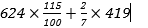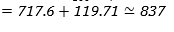Quantitative MCQ - 15 - Question 20

Directions (Q.16-20): What approximate value will come in place of question mark (?) in the following questions? (You are not expected to calculate the exact value.)

9654÷21+7638÷44= ?

Detailed Solution for Quantitative MCQ - 15 - Question 20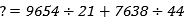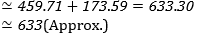Quantitative MCQ - 15 - Question 21

Directions (Q.21-25): In the following number series only one number is wrong. Find out wrong number.

71,  90, 128, 185, 261, 365

Detailed Solution for Quantitative MCQ - 15 - Question 21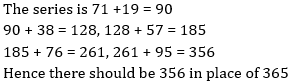Quantitative MCQ - 15 - Question 22

Directions (Q.21-25): In the following number series only one number is wrong. Find out wrong number.

6, 7, 16, 41, 90, 154, 292

Detailed Solution for Quantitative MCQ - 15 - Question 22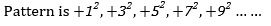Quantitative MCQ - 15 - Question 23

Directions (Q.21-25): In the following number series only one number is wrong. Find out wrong number.

4, 2.5,    3.5, 6.5, 15.5, 41.25, 126.75

Detailed Solution for Quantitative MCQ - 15 - Question 23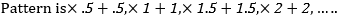Quantitative MCQ - 15 - Question 24

Directions (Q.21-25): In the following number series only one number is wrong. Find out wrong number.

5,    7, 16, 57, 244, 1245, 7506

Detailed Solution for Quantitative MCQ - 15 - Question 24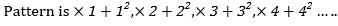Quantitative MCQ - 15 - Question 25

Directions (Q.21-25): In the following number series only one number is wrong. Find out wrong number.

1,       4,       25,       256,       3125,       46656,       823543

Detailed Solution for Quantitative MCQ - 15 - Question 25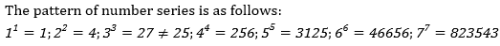Quantitative MCQ - 15 - Question 26

Weight of two persons A and B are in the ratio of 3 : 5. A’s weight increases by 20% and the total weight of A and B together becomes 80 kg, with an increase of 25%. By what percent did the weight of B increase?

Detailed Solution for Quantitative MCQ - 15 - Question 26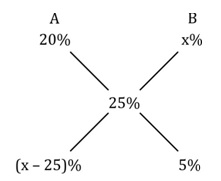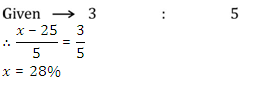Quantitative MCQ - 15 - Question 27

The ratio of the prices of two houses A and B was 4 : 5 last year. This year, the price of A is increased by 25 percent and that of B by Rs. 50,000. If their prices are now in the ratio 9 : 10, the price of A last year was:

Detailed Solution for Quantitative MCQ - 15 - Question 27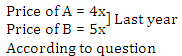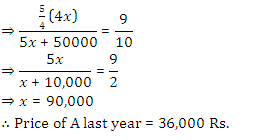= 360000

Quantitative MCQ - 15 - Question 28

A man borrows Rs. 6000 at 10% compound rate of interest. He pays back Rs. 2000 at the end of each year to clear his debt. The amount that he should pay to clear all his dues at the end of third year is:

Detailed Solution for Quantitative MCQ - 15 - Question 28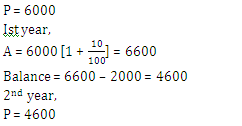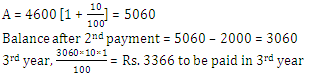Quantitative MCQ - 15 - Question 29

A vessel contains a mixture of liquid A and liquid B in the ratio 3 : 2. Five litres of mixture are taken out and replaced by liquid B. Thereafter, the ratio of liquid A and liquid B becomes 2 : 3. Find the quantity of liquid A in the mixture initially.

Detailed Solution for Quantitative MCQ - 15 - Question 29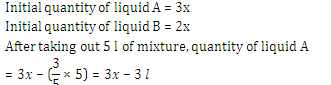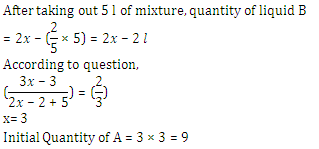Quantitative MCQ - 15 - Question 30

A man purchased 120 laptop tables at the rate of Rs. 80 per table. He spent Rs 280 on transportation, paid tax at the rate of 40 paise per table and paid Rs 72 to the coolie. If he wants to have a gain of 8%, the selling price per table must be (in Rs)

Detailed Solution for Quantitative MCQ - 15 - Question 30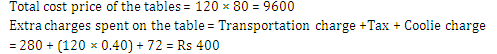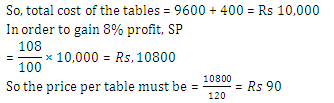## Quantitative Aptitude for Competitive Examinations

33 videos|34 docs|140 tests
 Use Code STAYHOME200 and get INR 200 additional OFF Use Coupon Code
Information about Quantitative MCQ - 15 Page
In this test you can find the Exam questions for Quantitative MCQ - 15 solved & explained in the simplest way possible. Besides giving Questions and answers for Quantitative MCQ - 15, EduRev gives you an ample number of Online tests for practice

## Quantitative Aptitude for Competitive Examinations

33 videos|34 docs|140 tests